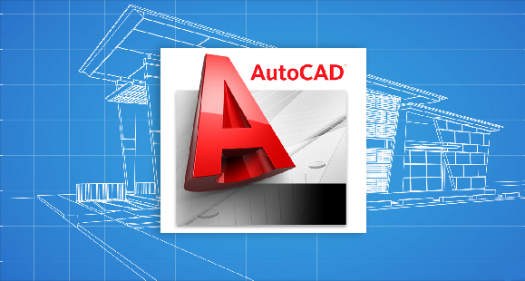# AutoCAD Level 1 Post- Assessment

12 Questions | Total Attempts: 176SettingsThis quiz will be used to assess your understanding of the AutoCAD software.

• 1.
By default, AutoCAD has the following workspaces
• A.

2D Drafting & Annotation

• B.

3D Modeling

• C.

My workspace

• D.

• 2.
What is the angle that you use to rotate the chair from point A to point B?
• A.

90

• B.

-90

• C.

-270

• D.

None

• 3.
One of the below options is wrong about type of Autocad drawing units. Which one is it?
• A.

Architectural

• B.

Engineering

• C.

Millimeter

• D.

Decimal

• 4.
Which of the following settings can be made for polar tracking?
• A.

Increment angle

• B.

• C.

Track using all polar angles settings

• 5.
In order to move an object, ________________
• A.

Select a base point,followed by a point to define where the object is moving to.

• B.

Use the Displacement option

• C.

Select object for grips, then move

• D.

All of the above

• 6.
Copy command also creates Multiples copies of an object. True/False?
• A.

True

• B.

False

• 7.
In order to get the below result using Object A, you have to set the number of rows and columns to:
• A.

4,1

• B.

4,2

• C.

4,3

• D.

4,4

• 8.
When using copy with grips, select Copy after you have selected an option, such as Move, Mirror, Rotate, Scale, or Stretch. True/False?
• A.

True

• B.

False

• 9.
What happens if you fillet these two lines with radius 0?
• A.

Cannot fillet

• B.

Sharp corner

• C.

None

• 10.
If you modify a dimension style, all dimensions using that style in the drawing updateautomatically. True/False?
• A.

True

• B.

False

• 11.
When you Explode a block, the geometry returns to its original properties. True/False?
• A.

True

• B.

False

• 12.
Which options are used to draw this polyline?
• A.

Line

• B.

Arc

• C.

Tamper

• D.

Width

Related TopicsBack to top